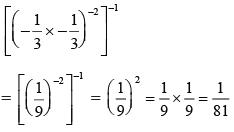# High Order Thinking Skills -2

## 20 Questions MCQ Test Mathematics Olympiad Class 7 | High Order Thinking Skills -2

Description
This mock test of High Order Thinking Skills -2 for Class 7 helps you for every Class 7 entrance exam. This contains 20 Multiple Choice Questions for Class 7 High Order Thinking Skills -2 (mcq) to study with solutions a complete question bank. The solved questions answers in this High Order Thinking Skills -2 quiz give you a good mix of easy questions and tough questions. Class 7 students definitely take this High Order Thinking Skills -2 exercise for a better result in the exam. You can find other High Order Thinking Skills -2 extra questions, long questions & short questions for Class 7 on EduRev as well by searching above.
QUESTION: 1

### The circumference of a circle is 176 cm. What is its diameter?

Solution:

Let r  be the radius of the circle.
2π r = 176.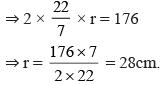Diameter = 28 × 2 = 56cm.

QUESTION: 2

### If the ratio between the length and perimeter of a rectangle is 1 : 3. What is the ratio of length and breadth of rectangle?

Solution: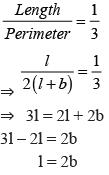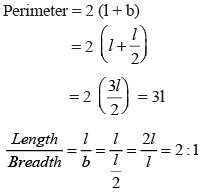QUESTION: 3

### Mr. Tiwari buys some stationaries for Rs. 237.35 and some books for Rs. 509.75. He gave a note of Rs. 1000 to the shopkeeper. How much money he gets from the shopkeeper?

Solution:

Required money
= 1000 – (237.35 + 509.75)
= 1000 – 747.10 = 252.90

QUESTION: 4

What is the product of additive inverse of (–17) and multiplicative inverse of 85?

Solution:

Additive inverse of –17 = 17 Multiplicative inverse of 85 = 1/85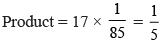QUESTION: 5

By what number should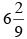be divided to obtain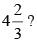Solution: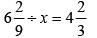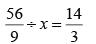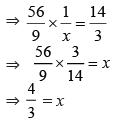QUESTION: 6

By selling apples at the rate of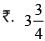per apple, a shopkeeper gets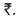420. How many apples does he sell?

Solution:

No. of apples =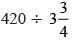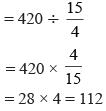QUESTION: 7

What is decimal equivalent of 7mm in kilometer?

Solution: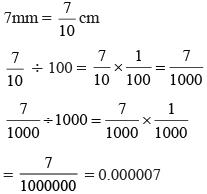QUESTION: 8

Write the simplified value of 72.09 – 26.83 + 18.96 – 37.24.

Solution:

72.09 – 26.83 + 18.96 – 37.24
= (72.09 + 18.96) – (26.83 + 37.24)
= 91.05 – 64.07 = 26.98

QUESTION: 9

What is the product of 0.8 × 0.008 × 8.8 ?

Solution:

0.8 × 0.008 × 8.8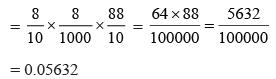QUESTION: 10

A bag contains 50 paise, 25 paise and 10 paise coins in the ratio 5 : 9 : 4 amounting to Rs. 206. What is the difference of the number of 50 paise and number of 25 paise coins?

Solution: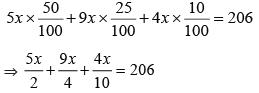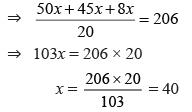No. of 50p coins = 5x = 5 × 40 = 200
No. of 25p coins = 9x = 9 × 40 = 360
Difference = 360 – 200 = 160

QUESTION: 11

​What is the perimeter of rectangle whose length is 40m and diagonal 41m?

Solution: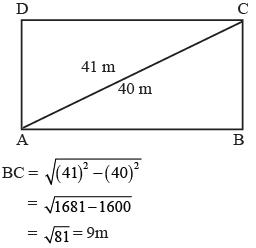QUESTION: 12

The cost of 6 dozen eggs is Rs 132. How much will 132 eggs cost?

Solution: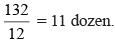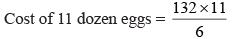= 22 × 11
= Rs. 242

QUESTION: 13

A number is increased by 10% and then decreased by 10%. What is the net increase or decrease percent?

Solution:

Let the number be 100.
After 10% increase, number = 100 + 10 = 110 Decrease 10% 110 – 10% of 110 = 110 – 11 = 99
Net decrease = 100 – 99 = 1%

QUESTION: 14

A sum of money lent at simple interest amounts to Rs. 3224 in 2 years and Rs. 4160 in 5 years. What is the rate of interest per amounts?

Solution:

Let the sum be P.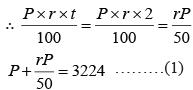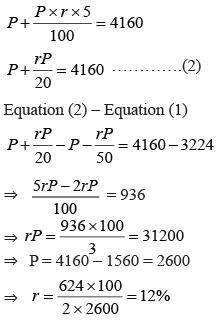QUESTION: 15

In the given figure, AB || CD, ∠ABO = 50°, ∠CDO = 40°. What is the measure of ∠BOD?​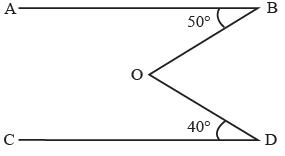Solution: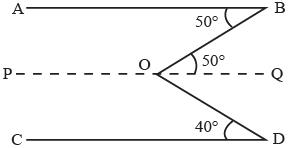AB || PQ ∠ABO = ∠BOQ = 50°
PQ || CD ∠ODC = ∠DOQ = 40°
∠BOD = 50° + 40° = 90°

QUESTION: 16

In the given figure x : y = 2 : 3, ∠ACD = 130°.
​What is the value of y?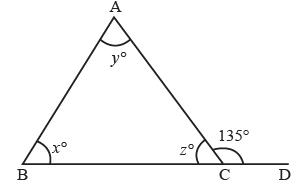Solution: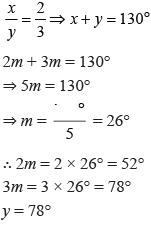QUESTION: 17

What is the area of a right triangle whose base is 1.2m and hypotenuse 3.7m?

Solution:

Area of right triangle =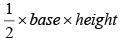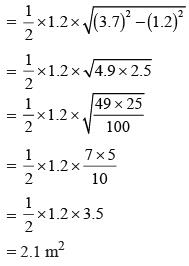QUESTION: 18

The circumference of a circle exceeds its diameter by 18cm. What is the radius of the circle?

Solution: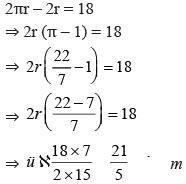QUESTION: 19

A rope makes 140 rounds of the circumference of a cylinder, the radius of whose base is 14cm. How many times can it go round a cylinder with radius 20cm?

Solution:

Length of rope = 2πr × 140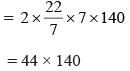Number of rounds =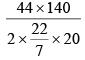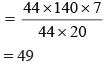QUESTION: 20

What is the value of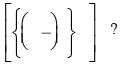Solution: